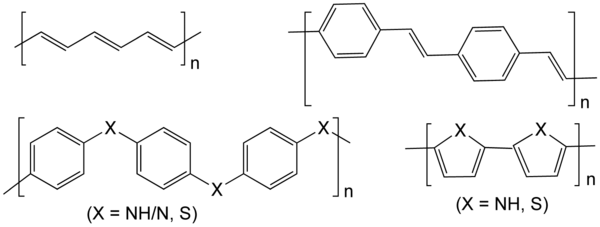## The 1D uniform electron gas under warm-dense matter conditions

The electronic structure of effectively one-dimensional conductive materials such as certain polymers or ultra-thin wires is relevant for electronics or nanotechnology. It determines chemical properties like reactivity and phase transitions including dimerization.

The electrochemical properties of conductive polymers give rise to interesting applications in various fields of electronics, such as energy storage and sensors. As is the case with conventional solid-state systems, an accurate study of such one-dimensional conductors requires a rigorous quantum mechanical treatment of the electronic correlation in the thermal system. Moreover, a simplified model system is chosen to reduce the complexity of the calculations.### Exact methods for the diagonlization of the hamiltonian

In this project we perform an exact diagonalization of the hamiltonian in second quantization for the 1D uniform electron gas at different temperatures and densities. This allows us to calculate the configuration’s free energy, as well as other macroscopic observables such as the expectation value of the internal energy. Numerical treatment is difficult since the resulting matrices grow exponentially with the system size.

Various optimizations allow for higher, still very limited, system sizes. These optimizations include the splitting of matrices into sub-blocks and the treatment of the largest of these blocks with sparse matrix algorithms.

The results from these efforts are used to benchmark more approximate theories such as coupled cluster or the random phase approximation.

### Matsubara frequency compression for the random phase approximation

The third pillar is the random phase approximation (RPA) in its finite temperature formulation. We choose RPA as it can straight-forwardly be applied to an infinite number of electrons by using integral rather than sums over states. In this setting, we aim to study the correlation contribution to the grand canonical potential for various densities and temperatures.

In the expression for the correlation contribution, a sum over infinite Matsubara frequencies poses a numerical challenge and we try to employ a Matsubara frequency compression scheme. Here, the sum over frequencies is approximated by an integral, which can then be evaluated numerically - as is the case for the other integrals over momenta. Finding optimized grids for an efficient quadrature of the integrals will determine the success of this project.